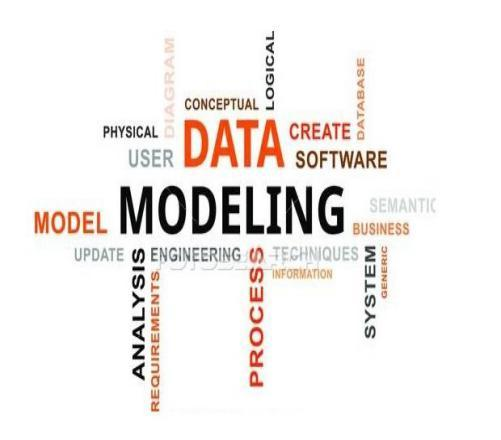# How Well Do You Know Your Data And Modelling?

10 Questions | Total Attempts: 11SettingsAn abstract model that organizes elements of data and standardizes how they relate to one another and to properties of the real world entities. Take this quiz to tell us how well you know your data and modelling.

• 1.
The term data model is used in two distinct but closely related senses. which of these two?
• A.

Geometry and formalization

• B.

Relationships and geometry

• C.

Abstract formalization and relationships.

• D.

None of the above

• 2.
Modelling may refer to which of the following?
• A.

Scientific model, a simplified and idealized understanding of physical systems

• B.

Physical model or plastic model, a physical representation in three dimensions of an object, such as a globe or model airplane

• C.

Conceptual model, a representation of a system using general rules and concepts

• D.

All of the above

• 3.
What is the main aim Data models?
• A.

The main aim of data models is analyze information by providing the definition and format of data.

• B.

The main aim of data models is to restructure information systems.

• C.

The main aim of data models is to support the development of information systems by providing the definition and format of data.

• D.

None of the above

• 4.
According to ANSI, data models is allows the ______ perspectives.
• A.

Two

• B.

Four

• C.

Five

• D.

Three

• 5.
One of the earliest pioneering works in modelling information system was done by who?
• A.

Charles Darwin

• B.

Young and Kent

• C.

Boyles and Kent

• D.

Elon Musk

• 6.
The earliest pioneering works in modelling information system was done in?
• A.

1958

• B.

1999

• C.

1934

• D.

1993

• 7.
How many type of Data models are there?
• A.

6 models

• B.

7 models

• C.

8 models

• D.

9 models

• 8.
Which of this is not a common model in Database model?
• A.

Star schema

• B.

Polar model

• C.

Network model

• D.

Relational model

• 9.
Which of the following  is a specification describing how a database is structured and used?
• A.

Data structure diagram

• B.

Semantic data model

• C.

Generic model model

• D.

Database model

• 10.
Which of the following  is the design of data for use in defining the target state and the subsequent planning needed to hit the target state?
• A.

Data architecture

• B.

Data structure

• C.

Data modelling

• D.

PatternBack to top